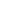Parameter StringsA number of Canvas methods take an optional second value called a Parameter String. These are used to specify or override options when executing the method.
 1How they work Parameters are provided as a list of items separated with spaces. Each parameter consists of a name (case insensitive) and a value. Often parameters may have a number of alternate names. For instance you can use the name 'rectangle' or 'rect' to specify a rectangular area. Both have the same effect. You enclose values in single quotes if they contain spaces themselves. If you want to indicate the presence of a single quote in a string, use two single quotes concatenated together (e.g. "don''t"). The following are examples of parameter strings as they might be used: ```ca.DrawText "Hello", "TextFont = 'Times' TextSize=12" ca.DrawShape "rect", "Pos = 0,0 Size=100,100 Inset=20" ca.DrawFile "pic.jpg", "Fit=True"```
 2Points Points are represented as two comma separated coordinates (x, y). If no value is provided the default point is 0,0. Coordinates may not be less than -32,000 or greater than +32,000. For example: ```ca.DrawShape "rect", "Pos = 0,0 Size=100,100 Inset=20"```
 3Rects Rects are represented as four comma separated integers (left, top, right, bottom). If no value is provided the default Rect is 0,0,0,0. Coordinates may not be less than -32,000 or greater than +32,000. For example: `ca.DrawShape "rect", "Rect=0,0,100,100 Inset=20"`
 4Colors Colors are represented either as literal names or as three or four floating point values (red, green, blue, alpha) each ranging between 0 and 100 percent. The alpha value is optional as it is not used when drawing and defaults to 100. For more details see the Specifying Colors section. For example all of the following draw red rectangles: ```ca.DrawShape "rect", "Rect=0,0,10,10 PaintColor=red" ca.DrawShape "rect", "Rect=0,0,10,10 PaintColor=100,0,0" ca.DrawShape "rect", "Rect=0,0,10,10 PaintColor=100.0,0.0,0.0,100.0" ca.DrawShape "rect", "Rect=0,0,10,10 PaintColor=0d255,0d0,0d0" ca.DrawShape "rect", "Rect=0,0,10,10 PaintColor=0xFF,0x00,0x00" ca.DrawShape "rect", "Rect=0,0,10,10 PaintColor=#ff0000"```
 5Booleans Boolean values may be indicated in a number of ways - 1, 0, true, false, yes, no, Y, n. If no value is provided then the default is false. For example all of the following are equivalent: ```ca.Dither = "true" ca.Dither = 1 ca.Dither = "yes" ca.Dither = true ca.Dither = "Y"```
 6Numbers Numbers may be provided as floating point or as integral values. They default to 0 if no value is provided. For example the following are equivalent: ```Width = 200 Width = 200.0 Width = "200"```